# Pixel grid

I started writing a post today about the ConvexImage property returned by regionprops. But I got sidetracked along the way, and this post turned into a description of how to overlay a pixel grid on an image. (I'll come back to the original ConvexImage idea in a future post.)

Here's what got me sidetracked. I made a tiny little binary image, and then I displayed it with high magnification because I wanted to show the individual pixels clearly as square regions.

bw = [...
0 0 0 1 0 0 0
0 0 1 0 1 0 0
0 1 0 0 0 1 0
1 1 1 1 1 1 1 ];

imshow(bw, 'InitialMagnification', 'fit')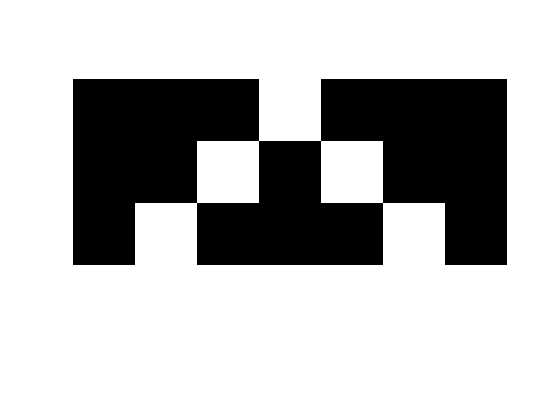Well, that doesn't look good! It's hard to see the individual pixels, and there's a whole row of white pixels at the bottom of the image that have disappeared into the background of the page. I decided that it would be nice to see a subtle but visible outline around each pixel. Here's what I have in mind:Let me show you how to do this in a fairly general fashion. When we're done, maybe I'll have something that would be worth posting to the MATLAB Central File Exchange.

First, I need to get a handle to the image object because I need some information about the x- and y-coordinates of the pixels. I'll use findobj to find the handle for the image object in the current figure.

h = findobj(gcf,'type','image');

The XData and YData properties of the image object determine where the image pixels lie in the axes data space.

xdata = get(h, 'XData')
xdata =

1     7


ydata = get(h, 'YData')
ydata =

1     4



This means:

• The x-coordinate of the center of the first image column is 1.0.
• The x-coordinate of the center of the last image column is 7.0.
• The y-coordinate of the center of the first image row is 1.0.
• The y-coordinate of the center of the last image row is 4.0.

Let's also grab the number of rows and columns of the image.

M = size(get(h,'CData'), 1);
N = size(get(h,'CData'), 2);

Reader challenge: Explain why I didn't use this code:

   [M,N] = size(get(h,'CData'));

Next I want to compute vectors containing the x- and y-locations of the pixel edges.

if M > 1
pixel_height = diff(ydata) / (M-1);
else
% Special case. Assume unit height.
pixel_height = 1;
end

if N > 1
pixel_width = diff(xdata) / (N-1);
else
% Special case. Assume unit width.
pixel_width = 1;
end

y_top = ydata(1) - (pixel_height/2);
y_bottom = ydata(2) + (pixel_height/2);
y = linspace(y_top, y_bottom, M+1)
y =

0.5000    1.5000    2.5000    3.5000    4.5000


x_left = xdata(1) - (pixel_width/2);
x_right = xdata(2) + (pixel_width/2);
x = linspace(x_left, x_right, N+1)
x =

0.5000    1.5000    2.5000    3.5000    4.5000    5.5000    6.5000    7.5000



Let me pause here and illustrate how we're going to use these numbers. Here's how to plot the vertical edge separating the 2nd and 3rd columns of pixels.

imshow(bw, 'InitialMagnification', 'fit')
hold on
plot([x(3) x(3)], [y(1) y(end)], 'r', 'LineWidth', 2)
hold off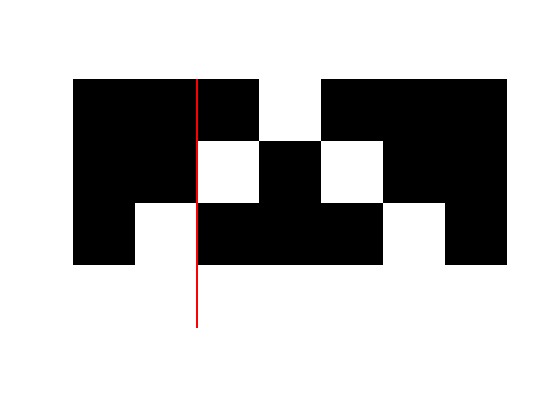We could plot the entire pixel grid by using N+1 vertical lines and M+1 horizontal lines. However, MATLAB graphics performance is usually better if you create fewer graphics objects. So I'm going to go to a little more effort in order to create only two line objects. One will snake up and down vertically(xv and yv); the other will snake left and right horizontally (xh and yh).

xv = zeros(1, 2*numel(x));
xv(1:2:end) = x;
xv(2:2:end) = x;

yv = repmat([y(1) ; y(end)], 1, numel(x));
yv(:,2:2:end) = flipud(yv(:,2:2:end));

xv = xv(:);
yv = yv(:);

imshow(bw, 'InitialMagnification', 'fit')
hold on
plot(xv, yv, 'r', 'LineWidth', 2, 'Clipping', 'off')
hold off
title('Vertical pixel edges')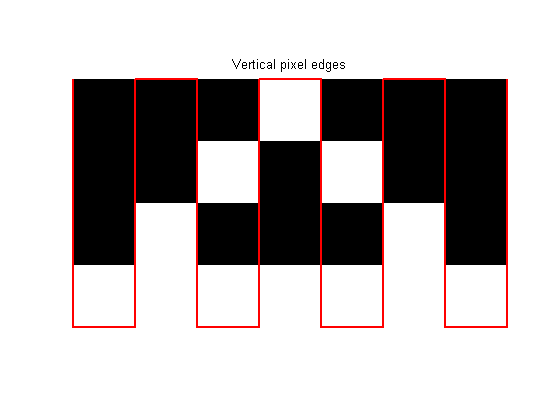yh = zeros(1, 2*numel(y));
yh(1:2:end) = y;
yh(2:2:end) = y;

xh = repmat([x(1) ; x(end)], 1, numel(y));
xh(:,2:2:end) = flipud(xh(:,2:2:end));

xh = xh(:);
yh = yh(:);

imshow(bw, 'InitialMagnification', 'fit')
hold on
plot(xh, yh, 'r', 'LineWidth', 2, 'Clipping', 'off')
hold off
title('Horizontal pixel edges')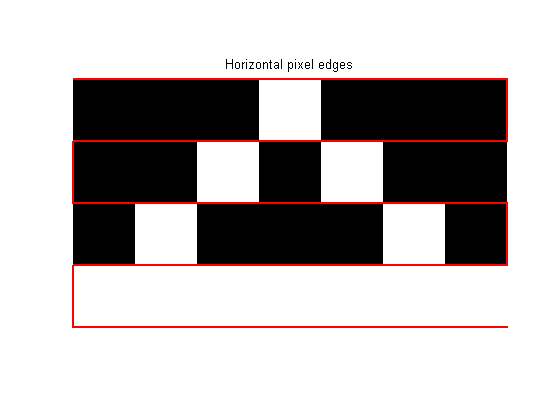To get all edges, superimpose the vertical and horizontal edges. Also, at this point I'm going to start using the low-level function line instead of plot. This is more robust and cuts out the need to call hold on and hold off.

h = imshow(bw, 'InitialMagnification', 'fit');
ax = ancestor(h, 'axes');
line('Parent', ax, 'XData', xh, 'YData', yh, ...
'Color', 'r', 'LineWidth', 2, 'Clipping', 'off');
line('Parent', ax, 'XData', xv, 'YData', yv, ...
'Color', 'r', 'LineWidth', 2, 'Clipping', 'off');
title('All pixel edges')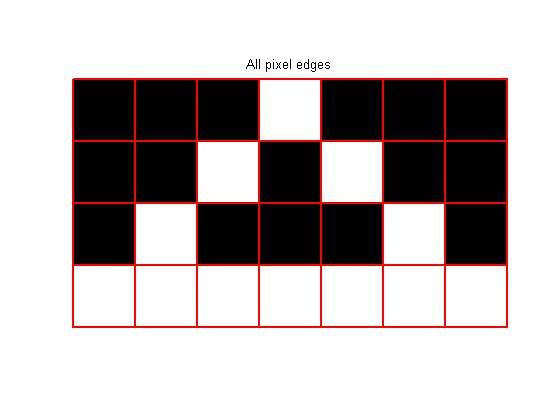But what if some of the pixels were red? We wouldn't be able to see the grid. I'm going to use the two-line superposition technique that I described way back on New Year's Day, 2007, and I'm going to switch to thinner lines using shades of gray.

The trick for drawing a line that's guaranteed to be visible against any background is to draw the line twice, using contrasting colors and a solid and a dashed line style.

dark = [.3 .3 .3];
light = [.8 .8 .8];
h = imshow(bw, 'InitialMagnification', 'fit');
ax = ancestor(h, 'axes');
line('Parent', ax, 'XData', xh, 'YData', yh, ...
'Color', dark, 'LineStyle', '-', 'Clipping', 'off');
line('Parent', ax, 'XData', xh, 'YData', yh, ...
'Color', light, 'LineStyle', '--', 'Clipping', 'off');
line('Parent', ax, 'XData', xv, 'YData', yv, ...
'Color', dark, 'LineStyle', '-', 'Clipping', 'off');
line('Parent', ax, 'XData', xv, 'YData', yv, ...
'Color', light, 'LineStyle', '--', 'Clipping', 'off');OK, readers, it's function design time. I think this technique could be encapsulated into a nice little utility function on the File Exchange. But before I go ahead and write it and submit, I'd like to open it up for discussion with you.

How about the implementation? The implementation above is basically the first thing I thought of; are there improvements?

How about the function name? I have one in mind, but what would you pick? How about the syntaxes? Input and output arguments? Options?